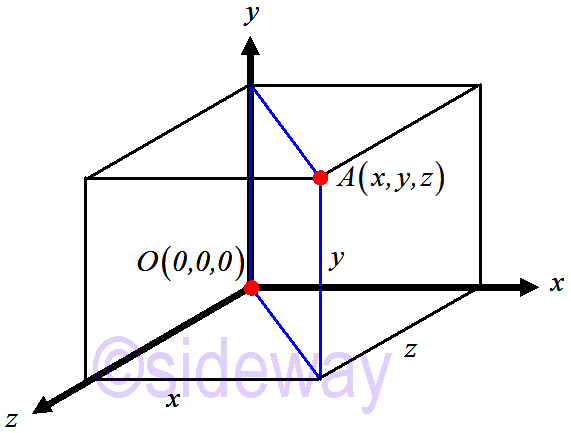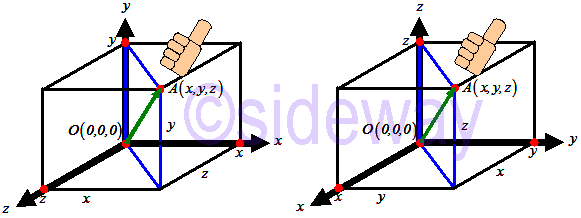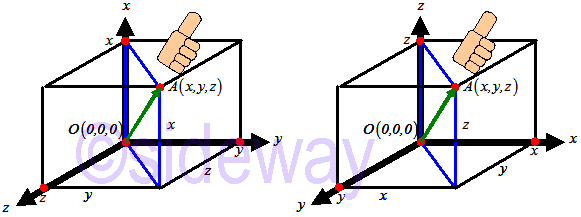output.to from Sideway
Draft for Information Only

# Content

`Vector Space Three-Dimensional Euclidean Space  Rectangular Cartesian Coordinate System   Rectangular Coordinate System Configuration   Rectangular Coordinate Configuration`

# Vector Space

The most common types of vector spaces are a three-dimensional Euclidean space, a two-dimensional subspace, and a one-dimensional subspace. But, vector can be extended to 𝑛-dimensional space.

## Three-Dimensional Euclidean Space

In a three-dimensional Euclidean space, a point can be represented in one-to-one correspondence by a real number triple with respect to a coordinate system.

### Rectangular Cartesian Coordinate SystemThe rectangular Cartesian coordinate system is the most common and important coordinate system used in vector manipulation. The three-dimensional space is constructed by three concurrent and mutually perpendicular lines. The set of all real numbers are one-to-one correspondence with the points of each line. The three lines are arranged with the point of concurrence corresponds to 0 and have a common unit of measurement. The three lines are called axes and are denoted as 𝑋1, 𝑋2, and 𝑋3 axes, or 𝑥, 𝑦, and 𝑧 axes. For convenient, the variables used to represent a point are usually designated by 𝑥1, 𝑥2, and 𝑥3, or 𝑥, 𝑦, and 𝑧. There always exists an ordered triple of real numbers (𝑥1,𝑥2,𝑥3) or (𝑥,𝑦,𝑧) corresponds to each point of the three-dimensional space and conversely. The triple of real number must be in correct order because the order of triple must correlate with the order of axes arrangement. Therefore both coordinate axes and triple variables are usually named in alphabetic and numeric order for convenient.

#### Rectangular Coordinate System Configuration

According to the arrangement of coordinate axes in space, a rectangular coordinate system can be divided into right-handed or left-handed configuration. The most commonly used rectangular coordinate system are right-handed configuration.The configuration of a rectangular coordinate system is determined by the arrangement of positive axes. Consider a vector from origin 𝑂(0,0,0) to any point 𝐴(𝑥,𝑦,𝑧) in the positive region. The rectangular coordinate system is said to be right-handed configuration if the order of coordinate axes arrangement following the order of coordinate component is in the direction of right hand fingers with the thumb pointing along with the vector 𝑂𝐴.Similarly, consider a vector from origin 𝑂(0,0,0) to any point 𝐴(𝑥,𝑦,𝑧) in the positive region. The rectangular coordinate system is said to be left-handed configuration if the order of coordinate axes arrangement following the order of coordinate component is in the direction of left hand fingers with the thumb pointing along with the vector 𝑂𝐴.

#### Rectangular Coordinate Configuration

```Rectangular Coordinate ConfigurationRight-Handed Rectangular Coordinate System : A rectangular Cartesian coordinate system is said to be right-handed only if the positive coordinate axes observed from outside to the origin is arranged following the order of coordinates in counterclockwise arrangement as the right hand fingers. Left-Handed Rectangular Coordinate System : A rectangular Cartesian coordinate system is said to be left-handed only if the positive coordinate axes observed from outside to the origin is arranged following the order of coordinates in clockwise arrangement as the right hand fingers. ```

ID: 200201002 Last Updated: 10/2/2020 Revision: 0 Ref:References

1. Robert C. Wrede, 2013, Introduction to Vector and Tensor Analysis
2. Daniel Fleisch, 2012, A Student’s Guide to Vectors and Tensors
3. Howard Anton, Chris Rorres, 2010, Elementary Linear Algebra: Applications VersionHome 5

Management

HBR 3

Information

Recreation

Culture

Chinese 1097

English 337

Computer

Hardware 149

Software

Application 198

Digitization 105

Numeric 19

Programming

Web 283

Unicode 494

HTML 65

CSS 58

ASP.NET 66

OS 389

Python 17

Knowledge

Mathematics

Algebra 25

Geometry 18

Calculus 67

Engineering

Mechanical

Rigid Bodies

Statics 92

Dynamics 37

Control

Physics

Electric 27